# 十六、比较两个样本

``````patients = Table.read_table('breast-cancer.csv').drop('ID')
shuffled_patients = patients.sample(with_replacement=False)
training_set = shuffled_patients.take(np.arange(341))
test_set  = shuffled_patients.take(np.arange(341, 683))
training_set
``````
Clump Thickness Uniformity of Cell Size Uniformity of Cell Shape Marginal Adhesion Single Epithelial Cell Size Bare Nuclei Bland Chromatin Normal Nucleoli Mitoses Class
5 1 1 1 2 1 2 1 1 0
5 1 1 1 1 1 1 1 1 0
4 1 1 1 2 1 1 1 1 0
5 1 2 1 2 1 3 1 1 0
4 10 8 5 4 1 10 1 1 1
7 2 4 1 3 4 3 3 1 1
9 4 5 10 6 10 4 8 1 1
3 1 1 1 2 2 3 1 1 0
3 2 1 1 2 1 2 2 1 0
6 3 3 5 3 10 3 5 3 0

（省略了 331 行）

``````training_cellsize = training_set.select('Class', 'Uniformity of Cell Size').relabel(1, 'Uniformity')
training_cellsize
``````
Class Uniformity
0 1
0 1
0 1
0 1
1 10
1 2
1 4
0 1
0 2
0 3

（省略了 331 行）

`Class``Uniformity`列显示为数字，但他们真的都是类别值。 这些类别是“癌症”（1）和“非癌症”（0）。 `Uniformity`为 1-10，但是这些标签是由人确定的，他们也可能有十个标签，如“非常一致”，“不一致”等等。 （一致性的 2 不一定是 1 的两倍。）所以我们比较两个类别分布，每个分类一个。

``````training_counts = training_cellsize.pivot('Class', 'Uniformity')
training_counts
``````
Uniformity 0 1
1 181 3
2 21 2
3 16 15
4 4 18
5 0 17
6 0 8
7 0 8
8 1 13
9 1 4
10 0 29

``````np.sum(training_counts.column('0'))
224
``````

``````def proportions(array):
return array/np.sum(array)
training_dists = training_counts.select(0).with_columns(
'0', proportions(training_counts.column('0')),
'1', proportions(training_counts.column('1'))
)
training_dists.barh('Uniformity')
``````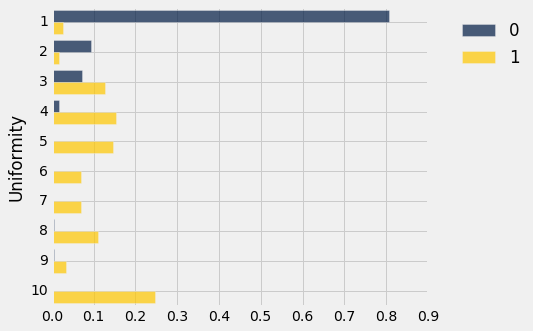``````test_set.take(np.arange(4)).column('Uniformity of Cell Size') > 3
array([ True, False, False, False], dtype=bool)
``````

``````classification = test_set.column('Uniformity of Cell Size') > 3

count_equal(classification, test_set.column('Class'))/test_set.num_rows
0.935672514619883
``````

## 两个类别分布

``````classes = Table().with_columns(
'Class', make_array(0, 1),
'Class Label', make_array('Not Cancer', 'Cancer')
)
patients = patients.drop('Class').relabel('Class Label', 'Class')
mitoses = patients.select('Class', 'Mitoses')
mitoses
``````
Class Mitoses
Not Cancer 1
Not Cancer 1
Not Cancer 1
Not Cancer 1
Not Cancer 1
Not Cancer 1
Not Cancer 1
Not Cancer 5
Not Cancer 1
Not Cancer 1

（省略了 673 行）

``````counts = mitoses.pivot('Class', 'Mitoses')
counts
``````
Mitoses Cancer Not Cancer
1 132 431
2 27 8
3 31 2
4 12 0
5 5 1
6 3 0
7 8 1
8 7 1
10 14 0
``````dists = counts.select(0).with_columns(
'Cancer', proportions(counts.column(1)),
'Not Cancer', proportions(counts.column(2))

)
dists.barh(0)
``````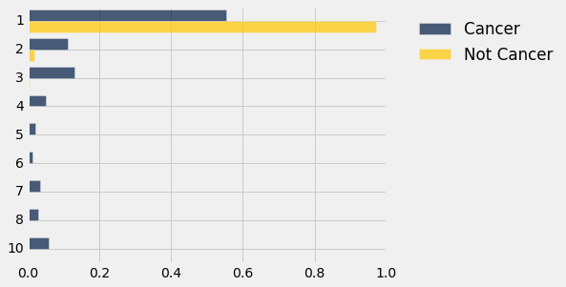### 假设

``````mitoses
``````
Class Mitoses
Not Cancer 1
Not Cancer 1
Not Cancer 1
Not Cancer 1
Not Cancer 1
Not Cancer 1
Not Cancer 1
Not Cancer 5
Not Cancer 1
Not Cancer 1

（省略了 673 行）

### 随机排列

``````shuffled_mitoses = mitoses.select('Mitoses').sample(with_replacement=False).column(0)
shuffled_mitoses.item(0)
1
``````

``````mitoses = mitoses.with_column('Shuffled Mitoses', shuffled_mitoses)
mitoses
``````
Class Mitoses Shuffled Mitoses
Not Cancer 1 1
Not Cancer 1 1
Not Cancer 1 1
Not Cancer 1 1
Not Cancer 1 7
Not Cancer 1 1
Not Cancer 1 1
Not Cancer 5 3
Not Cancer 1 1
Not Cancer 1 2

（省略了 673 行）

``````shuffled = mitoses.select('Class', 'Shuffled Mitoses')

shuffled_counts = shuffled.pivot('Class', 'Shuffled Mitoses')

shuffled_counts
``````
Shuffled Mitoses Cancer Not Cancer
1 199 364
2 12 23
3 12 21
4 5 7
5 2 4
6 0 3
7 3 6
8 3 5
10 3 11

``````shuffled_dists = shuffled_counts.select(0).with_columns(
'Cancer', proportions(shuffled_counts.column(1)),
'Not Cancer', proportions(shuffled_counts.column(2))
)
shuffled_dists.barh(0)
````````````dists.barh(0)
``````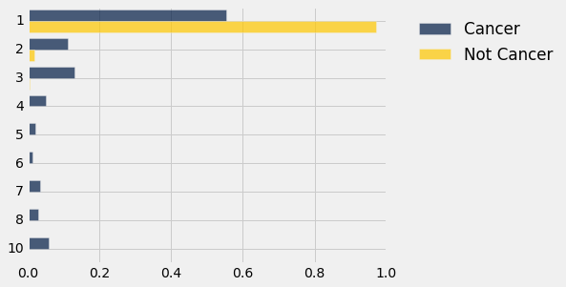### 检验统计量：总变异距离

``````def tvd(dist1, dist2):
return 0.5*(np.sum(np.abs(dist1 - dist2)))
``````

``````observed_tvd = tvd(dists.column(1), dists.column(2))
observed_tvd
0.41841946549059517
``````

``````tvd(shuffled_dists.column(1), shuffled_dists.column(2))
0.022173847487655045
``````

``````shuffled_mitoses = mitoses.select('Mitoses').sample(with_replacement=False).column(0)

shuffled = mitoses.select('Class').with_column('Shuffled Mitoses', shuffled_mitoses)

shuffled_counts = shuffled.pivot('Class', 'Shuffled Mitoses')

tvd(proportions(shuffled_counts.column(1)), proportions(shuffled_counts.column(2)))
0.039937426966715643
``````

### 原假设下 TVD 的经验分布

``````repetitions = 5000
tvds = make_array()
for i in np.arange(repetitions):
shuffled_mitoses = mitoses.select('Mitoses').sample(with_replacement=False).column(0)
shuffled = mitoses.select('Class').with_column('Shuffled Mitoses', shuffled_mitoses)
shuffled_counts = shuffled.pivot('Class', 'Shuffled Mitoses')
new_tvd = tvd(proportions(shuffled_counts.column(1)), proportions(shuffled_counts.column(2)))
tvds = np.append(tvds, new_tvd)

Table().with_column('TVD', tvds).hist(bins=20)
plots.title('Empirical Distribution Under the Null')
print('Observed TVD:', observed_tvd)
Observed TVD: 0.418419465491
``````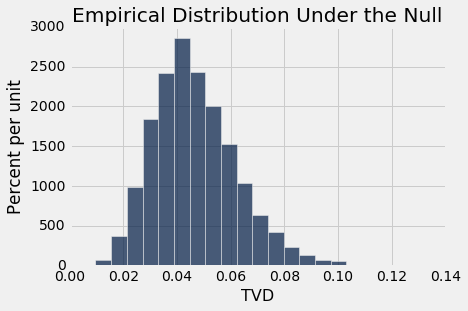### 两个类别分布的相等性的排列检验

``````def permutation_test_tvd(table, variable, classes, repetitions):

"""Test whether a categorical variable is independent of classes:
table: name of table containing the sample
variable: label of column containing categorical variable whose distribution is of interest
classes: label of column containing binary class data
repetitions: number of random permutations"""

# Find the tvd between the distributions of variable in the two classes
counts = table.select(classes, variable).pivot(classes, variable)
observed_tvd = tvd(proportions(counts.column(1)), proportions(counts.column(2)))

# Assuming the null is true, randomly permute the variable and collect all the new tvd's
tvds = make_array()
for i in np.arange(repetitions):
shuffled_var = table.select(variable).sample(with_replacement=False).column(0)
shuffled = table.select(classes).with_column('Shuffled Variable', shuffled_var)
shuffled_counts = shuffled.pivot(classes, 'Shuffled Variable')
new_tvd =tvd(proportions(shuffled_counts.column(1)), proportions(shuffled_counts.column(2)))
tvds = np.append(tvds, new_tvd)

# Find the empirical P-value:
emp_p = np.count_nonzero(tvds >= observed_tvd)/repetitions

# Draw the empirical histogram of the tvd's generated under the null,
# and compare with the value observed in the original sample
Table().with_column('TVD', tvds).hist(bins=20)
plots.title('Empirical Distribution Under the Null')
print('Observed TVD:', observed_tvd)
print('Empirical P-value:', emp_p)
permutation_test_tvd(patients, 'Clump Thickness', 'Class', 5000)
Observed TVD: 0.638310905047
Empirical P-value: 0.0
``````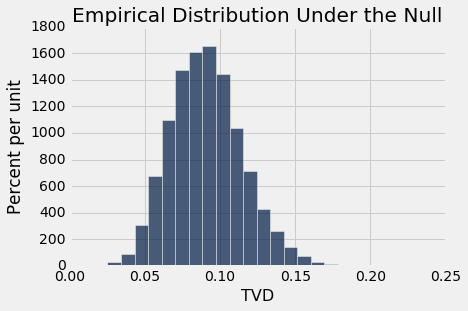## A/B 测试

### 吸烟者和不吸烟者

``````baby = Table.read_table('baby.csv')
baby
``````
Birth Weight Gestational Days Maternal Age Maternal Height Maternal Pregnancy Weight Maternal Smoker
120 284 27 62 100 False
113 282 33 64 135 False
128 279 28 64 115 True
108 282 23 67 125 True
136 286 25 62 93 False
138 244 33 62 178 False
132 245 23 65 140 False
120 289 25 62 125 False
143 299 30 66 136 True
140 351 27 68 120 False

（省略了 1164 行）

``````weight_smoke = baby.select('Birth Weight', 'Maternal Smoker')
weight_smoke.group('Maternal Smoker')
``````
Maternal Smoker count
False 715
True 459

``````nonsmokers = baby.where('Maternal Smoker', are.equal_to(False))
nonsmokers.hist('Birth Weight', bins=np.arange(40, 181, 5), unit='ounce')
``````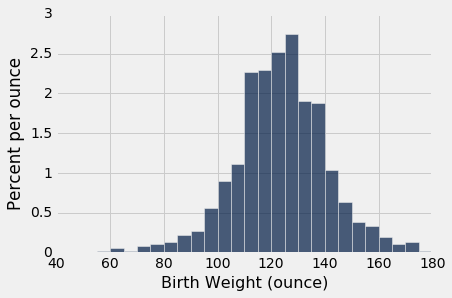``````smokers = baby.where('Maternal Smoker', are.equal_to(True))
smokers.hist('Birth Weight', bins=np.arange(40, 181, 5), unit='ounce')
``````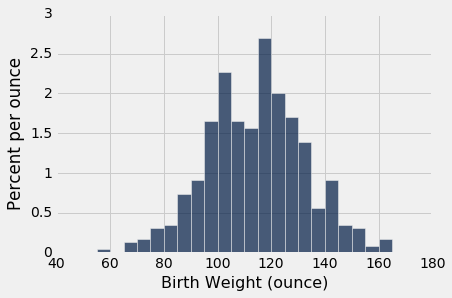``````means_table = weight_smoke.group('Maternal Smoker', np.mean)
means_table
``````
Maternal Smoker Birth Weight mean
False 123.085
True 113.819
``````nonsmokers_mean = means_table.column(1).item(0)
smokers_mean = means_table.column(1).item(1)
nonsmokers_mean - smokers_mean
9.266142572024918
``````

## 排列检验

``````def permutation_test_means(table, variable, classes, repetitions):

"""Test whether two numerical samples
come from the same underlying distribution,
using the absolute difference between the means.
table: name of table containing the sample
variable: label of column containing the numerical variable
classes: label of column containing names of the two samples
repetitions: number of random permutations"""

t = table.select(variable, classes)

# Find the observed test statistic
means_table = t.group(classes, np.mean)
obs_stat = abs(means_table.column(1).item(0) - means_table.column(1).item(1))

# Assuming the null is true, randomly permute the variable
# and collect all the generated test statistics
stats = make_array()
for i in np.arange(repetitions):
shuffled_var = t.select(variable).sample(with_replacement=False).column(0)
shuffled = t.select(classes).with_column('Shuffled Variable', shuffled_var)
m_tbl = shuffled.group(classes, np.mean)
new_stat = abs(m_tbl.column(1).item(0) - m_tbl.column(1).item(1))
stats = np.append(stats, new_stat)

# Find the empirical P-value:
emp_p = np.count_nonzero(stats >= obs_stat)/repetitions

# Draw the empirical histogram of the tvd's generated under the null,
# and compare with the value observed in the original sample
Table().with_column('Test Statistic', stats).hist(bins=20)
plots.title('Empirical Distribution Under the Null')
print('Observed statistic:', obs_stat)
print('Empirical P-value:', emp_p)
permutation_test_means(baby, 'Birth Weight', 'Maternal Smoker', 5000)
Observed statistic: 9.266142572024918
Empirical P-value: 0.0
``````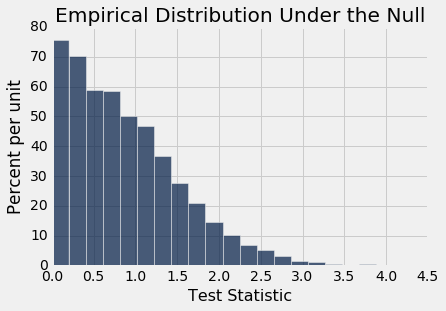## 差值的自举置信区间

• 表名称，它包含原始样本中的数据
• 列标签，它包含数值变量
• 列标签，它包含两个样本的名称
• 自举的重复次数

``````def bootstrap_ci_means(table, variable, classes, repetitions):

"""Bootstrap approximate 95% confidence interval
for the difference between the means of the two classes
in the population"""

t = table.select(variable, classes)

mean_diffs = make_array()
for i in np.arange(repetitions):
bootstrap_sample = t.sample()
m_tbl = bootstrap_sample.group(classes, np.mean)
new_stat = m_tbl.column(1).item(0) - m_tbl.column(1).item(1)
mean_diffs = np.append(mean_diffs, new_stat)

left = percentile(2.5, mean_diffs)
right = percentile(97.5, mean_diffs)

# Find the observed test statistic
means_table = t.group(classes, np.mean)
obs_stat = means_table.column(1).item(0) - means_table.column(1).item(1)

Table().with_column('Difference Between Means', mean_diffs).hist(bins=20)
plots.plot(make_array(left, right), make_array(0, 0), color='yellow', lw=8)
print('Observed difference between means:', obs_stat)
print('Approximate 95% CI for the difference between means:')
print(left, 'to', right)
bootstrap_ci_means(baby, 'Birth Weight', 'Maternal Smoker', 5000)
Observed difference between means: 9.266142572024918
Approximate 95% CI for the difference between means:
7.23940878698 to 11.3907887554
``````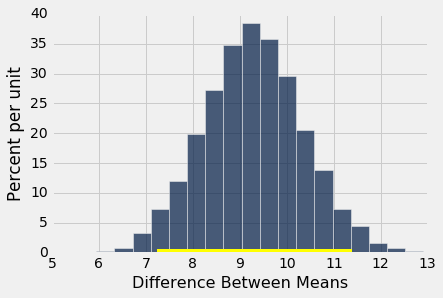``````bootstrap_ci_means(baby, 'Maternal Age', 'Maternal Smoker', 5000)
Observed difference between means: 0.8076725017901509
Approximate 95% CI for the difference between means:
0.154278698588 to 1.4701157656
``````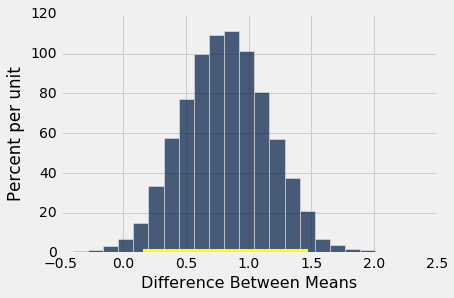``````bootstrap_ci_means(baby, 'Maternal Height', 'Maternal Smoker', 5000)
Observed difference between means: 0.09058914941267915
Approximate 95% CI for the difference between means:
-0.390841928035 to 0.204388297872
``````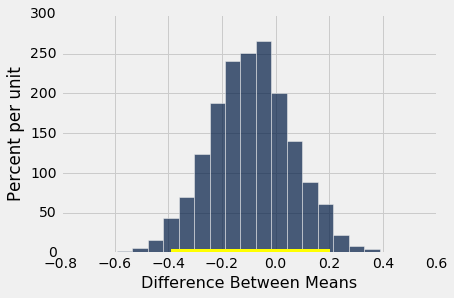## 因果

### 治疗慢性背痛：RCT

``````bta = Table.read_table('bta.csv')
bta
``````
Group Result
Control 1
Control 1
Control 0
Control 0
Control 0
Control 0
Control 0
Control 0
Control 0
Control 0

（省略了 21 行）

``````bta.group('Group', np.mean)
``````
Group Result mean
Control 0.125
Treatment 0.6

### 潜在的结果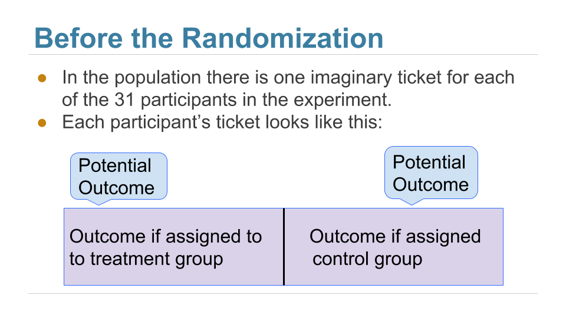`observed_outcomes`表收集每个患者潜在结果的信息，每张“票”的未观察的一半留空。 （这只是考虑`bta`表的另一种方式，它载有的信息相同。）

``````observed_outcomes = Table.read_table("observed_outcomes.csv")
observed_outcomes.show()
``````
Group Outcome if assigned treatment Outcome if assigned control
Control Unknown 1
Control Unknown 1
Control Unknown 0
Control Unknown 0
Control Unknown 0
Control Unknown 0
Control Unknown 0
Control Unknown 0
Control Unknown 0
Control Unknown 0
Control Unknown 0
Control Unknown 0
Control Unknown 0
Control Unknown 0
Control Unknown 0
Control Unknown 0
Treatment 1 Unknown
Treatment 1 Unknown
Treatment 1 Unknown
Treatment 1 Unknown
Treatment 1 Unknown
Treatment 1 Unknown
Treatment 1 Unknown
Treatment 1 Unknown
Treatment 1 Unknown
Treatment 0 Unknown
Treatment 0 Unknown
Treatment 0 Unknown
Treatment 0 Unknown
Treatment 0 Unknown
Treatment 0 Unknown

### 假设检验

``````def permutation_test_means(table, variable, classes, repetitions):

"""Test whether two numerical samples
come from the same underlying distribution,
using the absolute difference between the means.
table: name of table containing the sample
variable: label of column containing the numerical variable
classes: label of column containing names of the two samples
repetitions: number of random permutations"""

t = table.select(variable, classes)

# Find the observed test statistic
means_table = t.group(classes, np.mean)
obs_stat = abs(means_table.column(1).item(0) - means_table.column(1).item(1))

# Assuming the null is true, randomly permute the variable
# and collect all the generated test statistics
stats = make_array()
for i in np.arange(repetitions):
shuffled_var = t.select(variable).sample(with_replacement=False).column(0)
shuffled = t.select(classes).with_column('Shuffled Variable', shuffled_var)
m_tbl = shuffled.group(classes, np.mean)
new_stat = abs(m_tbl.column(1).item(0) - m_tbl.column(1).item(1))
stats = np.append(stats, new_stat)

# Find the empirical P-value:
emp_p = np.count_nonzero(stats >= obs_stat)/repetitions

# Draw the empirical histogram of the tvd's generated under the null,
# and compare with the value observed in the original sample
Table().with_column('Test Statistic', stats).hist()
plots.title('Empirical Distribution Under the Null')
print('Observed statistic:', obs_stat)
print('Empirical P-value:', emp_p)
permutation_test_means(bta, 'Result', 'Group', 20000)
Observed statistic: 0.475
Empirical P-value: 0.00965
``````### 效果的置信区间

``````group_means = bta.group('Group', np.mean)
group_means
``````
Group Result mean
Control 0.125
Treatment 0.6
``````group_means.column(1).item(0) - group_means.column(1).item(1)
-0.475
``````

``````def bootstrap_ci_means(table, variable, classes, repetitions):

"""Bootstrap approximate 95% confidence interval
for the difference between the means of the two classes
in the population"""

t = table.select(variable, classes)

mean_diffs = make_array()
for i in np.arange(repetitions):
bootstrap_sample = t.sample()
m_tbl = bootstrap_sample.group(classes, np.mean)
new_stat = m_tbl.column(1).item(0) - m_tbl.column(1).item(1)
mean_diffs = np.append(mean_diffs, new_stat)

left = percentile(2.5, mean_diffs)
right = percentile(97.5, mean_diffs)

# Find the observed test statistic
means_table = t.group(classes, np.mean)
obs_stat = means_table.column(1).item(0) - means_table.column(1).item(1)

Table().with_column('Difference Between Means', mean_diffs).hist(bins=20)
plots.plot(make_array(left, right), make_array(0, 0), color='yellow', lw=8)
print('Observed difference between means:', obs_stat)
print('Approximate 95% CI for the difference between means:')
print(left, 'to', right)
bootstrap_ci_means(bta, 'Result', 'Group', 20000)
Observed difference between means: -0.475
Approximate 95% CI for the difference between means:
-0.759090909091 to -0.162393162393
``````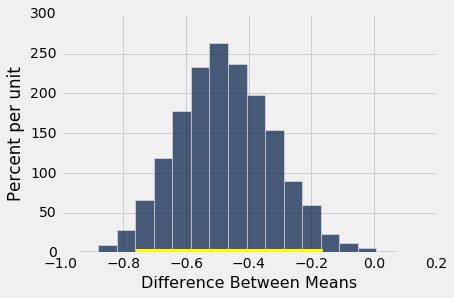### 元分析

2011 年，一组研究人员对实验的研究进行了元分析。也就是说，他们确定了所有被痛治疗的可用研究，并总结了整理后的结果。